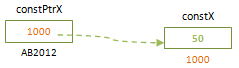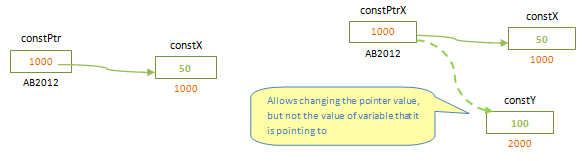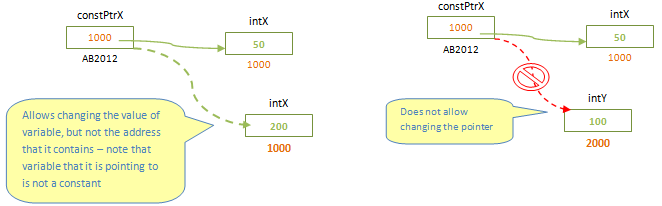Constant Pointers in C Programming

Usually variables hold values and those values can be changed as per the requirement. But we have constant variables, whose value is constant throughout the program. Can we assign pointers to such constant variables? Since constant variables are also variables and they occupy some memory in the system, we can define a pointer which points to them.

const int *constPtrX =constX;Since the value of constant variable constX cannot be changed, we cannot change the value of this variable using constant pointer too. We can see above that pointer is declared in the same way as we declare a constant variable. It is an integer pointer to a constant variable. Hence it holds the same feature, except that it holds the address of that variable.

Does the following declaration is same as above declaration ?

int const *constPtrX =constX;Yes, both these declarations are equivalent. Both are pointers to constant integer variable. What happens when we declare a pointer as shown below? Are they equivalent ?

int *const constPtrX =constX;

This is not equivalent to other two declarations. Here the pointer is not pointing to constant variable; rather pointer itself is a constant. That means the value pointed by the pointer can be changed but we cannot change the address of the pointer constPtrX. For example, suppose *constPtrX = 50. If we want to change the value of intX, then we can change it to any other integer value and pointer constPtrX will still hold same address 1000 (address of the variable). Suppose now we want to assign another variable intY to this pointer. Will this be possible with above declaration? Since pointer itself is a constant, we cannot change the address that it has. That means here pointer acts as a constant variable. We cannot change its value. We cannot perform pointer arithmetic on constant pointers.